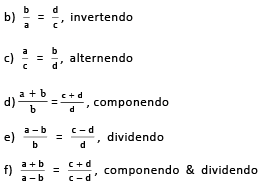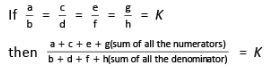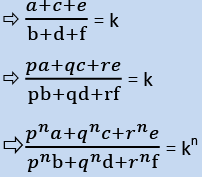Courses

Important Formula: Ratio & Proportion Notes | Study Quantitative Aptitude (Quant) - CAT

CAT: Important Formula: Ratio & Proportion Notes | Study Quantitative Aptitude (Quant) - CAT

The document Important Formula: Ratio & Proportion Notes | Study Quantitative Aptitude (Quant) - CAT is a part of the CAT Course Quantitative Aptitude (Quant).
All you need of CAT at this link: CAT

Ratio and Proportions is one of the easiest concepts in CAT. It is just an extension of high school mathematics.

• Questions from this concept are mostly asked in conjunction with other concepts like similar triangles, mixtures and alligations.
• Hence fundamentals of this concept are important not just from a stand-alone perspective, but also to answer questions from other concept
1. Ratio is the comparison between similar types of quantities; it is an abstract quantity and does not have any units.
2. If a/b = c/d , then a, b, c, d are said to be in proportion.
3. If a/b = c/d, then
If a, b, x are positive, then
If a>b, then a+x/b+x <a/b
If a<b,then a+x/b+x >a/b
If a>b,then a−x/b−x >a/b
If a<b,then a−x/b-x<a/b
4. If a/p = b/q = c/r = d/s = ..., then a:b:c:d:...= p:q:r:s:...
(a) a × d = c × b5. If a, b, x are positive, then
If a>b, then a+x/b+x < a/b
If a<b, then a+x/b+x > a/b
If a>b, then a−x/b−x > a/b
If a<b, then a−x/b-x < a/b
6. If a/p= b/q = c/r =d/s =...,then a:b:c:d:...=p:q:r:s:...

7.8. Types of ratios:
Duplicate Ratio of a:b is a2 :b2
Sub-duplicate ratio of a:b is Sqrt(a): Sqrt(b)
Triplicate Ratio of a:b is a3 :b3
Sub-triplicate ratio of a : b is a1/3 : b1/3

Product of proportions:

If a:b = c:d is a proportion, then

• Product of extremes = product of means i.e., ad = bc
• Denominator addition/subtraction: a:a+b = c:c+d and a:a-b = c:c-d
• a, b, c, d,.... are in continued proportion means, a:b = b:c = c:d = ....
• a:b = b:c then b is called mean proportional and b2 = ac
• The third proportional of two numbers, a and b, is c, such that, a:b = b:c ▪ d is fourth proportional to numbers a, b, c if a:b = c:d

Variations:

• If a ∝ b, provided c is constant and a ∝ c, provided b is constant, then a ∝ b x c, if all three of them are varying.
• If A and B are in a business for the same time, then Profit distribution ∝ Investment (Time is constant).
• If A and B are in a business with the same investment, then Profit distribution ∝ Time of investment (Investment is constant).
• Profit Distribution ∝ Investment × Time.

EduRev's Tip: If a/b = c/d = e/f = kGiven two variables x and y, y is (directly) proportional to x (x and y vary directly, or x and y are in direct variation) if there is a non-zero constant k such that y = kx. It is denoted by y ∝ x
Two variables are inversely proportional (or varying inversely, or in inverse variation, or in inverse proportion or reciprocal proportion) if there exists a nonzero constant k such that y = k/x.

The document Important Formula: Ratio & Proportion Notes | Study Quantitative Aptitude (Quant) - CAT is a part of the CAT Course Quantitative Aptitude (Quant).
All you need of CAT at this link: CATUse Code STAYHOME200 and get INR 200 additional OFF Use Coupon Code

Top Courses for CATQuantitative Aptitude (Quant)

134 videos|131 docs|131 tests

Top Courses for CATHow to Prepare for CAT

Read our guide to prepare for CAT which is created by Toppers & the best Teachers

Track your progress, build streaks, highlight & save important lessons and more!

,

,

,

,

,

,

,

,

,

,

,

,

,

,

,

,

,

,

,

,

,

;